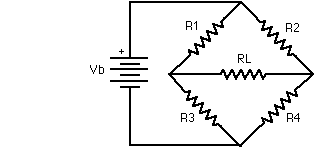# problem in finding effective resistance

Look to this figure:i have doubt in finding effective resistance b/w the terminals of this type of connections, during my school classes when I study to find effective resistances. When I study about wheatstone bridge. A method get me to find it, provided the relation $$frac{R_1}{R_3}=\frac{R_2}{R_4}$$ satisfied. Then there is no current through $R_L$ and circuit is simplified. But when the above relation does not satisfied, then how I find effective resistance? Is there any analytical method to find such solution or is this values only determine experimentally?

• Are you familiar with Thévenin? – jippie Jan 2 '16 at 16:29